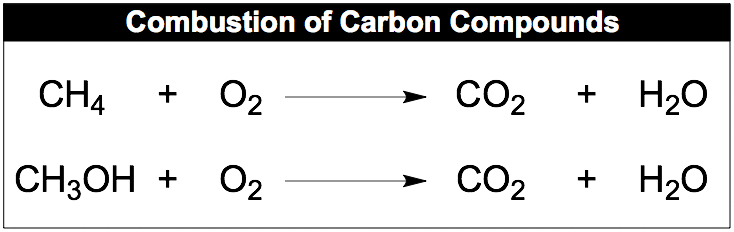Ch.3 - Chemical ReactionsWorksheetSee all chapters

# Combustion Analysis

See all sections
Sections
Empirical Formula
Molecular Formula
Combustion Analysis
Combustion Apparatus
Polyatomic Ions
Naming Ionic Compounds
Writing Ionic Compounds
Naming Ionic Hydrates
Naming Acids
Naming Molecular Compounds
Balancing Chemical Equations
Stoichiometry
Limiting Reagent
Percent Yield
Mass Percent
Functional Groups in Chemistry
Molecular Models
Ionic and Covalent Compounds
Metal Alloys

Under Combustion Analysis, the empirical formula of a compound is determined through a combustion reaction.

###### Combustion Analysis

Concept #1: Combustion AnalysisExample #1: A 0.2500 g sample contains carbon, hydrogen and oxygen and undergoes complete combustion to produce 0.3664 g of CO2 and 0.1500 g of H2O. What is the empirical formula of the compound?

Practice: Valproic acid, used to treat seizures, is composed of carbon, hydrogen and oxygen. A 0.165 g sample is combusted to produce 0.166 grams of water and 0.403 grams of carbon dioxide. If the molar mass is 144 g/mol, what is the molecular formula?

Practice: When hydrocarbons are burned in a limited amount of air, both CO and CO2 form. When 0.460 g of a hydrocarbon was burned in air, 0.477 g of CO, 0.749 g CO2, and 0.460 g of H2O were formed. What is the empirical formula of the compound?

Concept #2: Combustion of Non-Hydrocarbons

Example #2: A solvating agent (MW = 147.0 g/mol) that contains C, H, and Cl is used for spectroscopic processes. Determine its molecular formula when a 0.250 g sample creates 0.451 g CO2 and 0.0617 g of H2O upon combustion.

Practice: If a compound made of calcium, carbon, nitrogen undergoes combustion in oxygen to create 2.389 g CaO, 1.876 g CO2, and 3.921 g NO­2, determine its empirical formula.

Practice: The combustion of 4.16 grams of a compound which contains only C,H,O and F yields 7.7 g CO2 and 2.52 g H2O. Another sample of the compound with a mass of 3.63 g is found to contain 0.58 g F. What is the empirical formula of the compound?